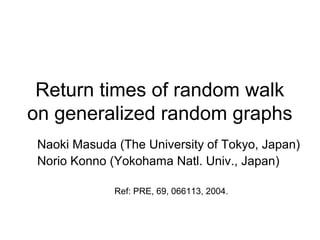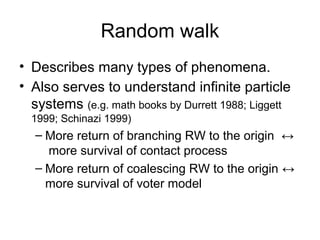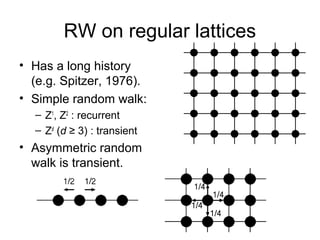Successfully reported this slideshow.

# Return times of random walk on generalized random graphs

1

Share×
1 of 18
1 of 18

# Return times of random walk on generalized random graphs

1

Share

Presentation slides for
Masuda and Konno. Physical Review E, 69, 066113 (2004).

Presentation slides for
Masuda and Konno. Physical Review E, 69, 066113 (2004).

## More Related Content

### Related Books

Free with a 14 day trial from Scribd

See all

### Related Audiobooks

Free with a 14 day trial from Scribd

See all

### Return times of random walk on generalized random graphs

1. 1. Return times of random walk on generalized random graphs Naoki Masuda (The University of Tokyo, Japan) Norio Konno (Yokohama Natl. Univ., Japan) Ref: PRE, 69, 066113, 2004.
2. 2. Random walk • Describes many types of phenomena. • Also serves to understand infinite particle systems (e.g. math books by Durrett 1988; Liggett 1999; Schinazi 1999) – More return of branching RW to the origin ↔ more survival of contact process – More return of coalescing RW to the origin ↔ more survival of voter model
3. 3. RW on regular lattices • Has a long history (e.g. Spitzer, 1976). • Simple random walk: – Z1, Z2 : recurrent – Zd (d ≥ 3) : transient • Asymmetric random walk is transient.
4. 4. RW on heterogeneous networks • Finite scale-free networks – Stationary density on node vi ≈ ki (e.g. Noh et al., 2004) • In this study, infinite tree-like networks with a general degree distribution
5. 5. RW on generalized random graphs (also called Galton-Watson trees) pk: degree distribution
6. 6. Recursion relation valid for small mean degrees • pk: degree distribution • qn: prob. that a walker returns to the origin at time 2n for the first time.
7. 7. Use of generating functions
8. 8. Expression of the return probability using the ‘moment’ generating function of the graph  zM Q ( z )  pk  k −1   Q ( z ) = z∑ ∑ Q ( z) ÷ = ,  k  Q ( z) k=1 k n=0 ∞ n ∞ ∞ M ( z ) ≡ ∑ mn z n , n=1 ∞ mn ≡ ∑ k=1 ( k −1) k n n−1 pk .
9. 9. Lagrange’s inversion formula z = w / f ( w) [ ] z d n  g [ w( z ) ] = g ( 0) + ∑n =1  n −1 g ′( u ) f ( u )  n!  du  u =0 ∞ n n −1 For our purpose, set w(z)=Q(z), f(w)=M(w)/w, g(w)=w.
10. 10. partition of n number of parts
11. 11. Partition of integers • Partitions of 5: (15), (132), (123), (122), (14), (23), (5) Young diagrams
12. 12. Restricted partition of integers • All the partition of 5 with 3 parts are: (1 23) and (122).
13. 13. Ex1) Cayley trees • pk=δk,d : homogeneous vertex degree 1 Q( z ) = d − (d − 1)Q( z ) (d − 1) n −1 qn = Dn −1 2 n −1 d → where d − d 2 − 4(d − 1) z Q( z ) = 2(d − 1) Cn Dn = n +1 2n : Catalan number • Consistent with well-known results (e.g. Spitzer, 1976).
14. 14. Ex2) Erdös-Renyí random graph • pk=λk e-λ/k! • Can be calculated up to a larger n as compared to the brute-force method. • A bit slower exponential decay than the Cayley tree case. qn numerical (5 ∙107 runs) theory Cayley trees (theory) n (time) d = λ =7 d = λ =10
15. 15. Ex3) scale-free networks • pk ∝ k −γ (k ≥ kmin) • Slower decay than the Cayley tree case. Effect of heterogeneity. qn numerical theory d=7 Cayley trees (theory) d=8 n (time) mean degree = 7.09
16. 16. Relation to other results • Generally, more heterogeneous vertex degree → more percolation, easier survival of CP and voter models. In scale-free networks with pk ∝ k−γ , critical point even disappears when γ≤ 3 – Percolation (Albert et al., 2000; Cohen et al., 2000) – CP (Pastor-Satorras & Vespignani, 2001; Eguíluz & Klemm, 2002). – SIR (Moreno et al., 2002) • For branching RW on GW trees (Madras & Schinazi, 1992; Schinazi, 1993), critical point for – global survival: λ1=1 ， – local survival: λ2=1/(1−τ) where qn ~ exp(−τn) ． • Our numerical results connect these two theoretical results.
17. 17. Conclusions • Explicit formula for return probability of RW on generalized random graphs. • Calculation up to a large time is possible as compared to the brute-force method. • Young diagrams appear. Relation between asymptotic distribution of Young diagrams and RW, CP, voter model, etc.?